# High School Math : Graphing Hyperbolas

## Example Questions

### Example Question #1 : Pre Calculus

A conic section is represented by the following equation: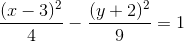Which of the following best describes this equation?

Possible Answers:

vertical parabola with vertex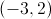and a vertical stretch factor ofvertical hyperbola with center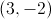and asymptotes with slopes ofand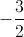vertical ellipse with centerand a major axis length of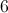horizontal hyperbola with centerand asymptotes with slopes ofand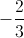horizontal hyperbola with center ofand asymptotes with slopes of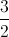andCorrect answer:

horizontal hyperbola with center ofand asymptotes with slopes ofandExplanation:

First, we need to make sure the conic section equation is in a form we recognize. Luckily, this equation is already in standard form: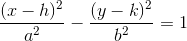The first step is to determine the type of conic section this equation represents. Because there are two squared variables (and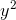), this equation cannot be a parabola.  Because the coefficients in front of the squared variables are different signs (i.e. one is negative and the other is positive), this equation must be a hyperbola, not an ellipse.

In a hyperbola, the squared term with a positive coefficient represents the direction in which the hyperbola opens. In other words, if theterm is positive, the hyperbola opens horizontally. If theterm is positive, the hyperbola opens vertically. Therefore, this is a horizontal hyperbola.

The center is always found at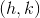, which in this case is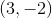.

That leaves only the asymptotes. For a hyperbola, the slopes of the asymptotes can be found by dividingby(remember to always put the vertical value,, above the horizontal value,). Remember that these slopes always come in pairs, with one being positive and the other being negative.

In this case,is 3 andis 2, so we get slopes ofand.

### All High School Math Resources